# Class 12 Mathematics: CBSE Sample Question Paper- Term I (2021-22)- 4 Notes | Study Mathematics (Maths) Class 12 - JEE

## JEE: Class 12 Mathematics: CBSE Sample Question Paper- Term I (2021-22)- 4 Notes | Study Mathematics (Maths) Class 12 - JEE

The document Class 12 Mathematics: CBSE Sample Question Paper- Term I (2021-22)- 4 Notes | Study Mathematics (Maths) Class 12 - JEE is a part of the JEE Course Mathematics (Maths) Class 12.
All you need of JEE at this link: JEE

Class-XII
Time: 90 Minutes
Max. Marks: 40

General Instructions :

1. This question paper contains three sections – A, B and C. Each part is compulsory.
2. Section - A has 20 MCQs, attempt any 16 out of 20.
3. Section - B has 20 MCQs, attempt any 16 out of 20.
4. Section - C has 10 MCQs, attempt any 8 out of 10.
5. There is no negative marking.
6. All questions carry equal marks

Section - A

Q.1: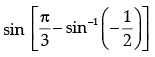is equal to:
(a) 1/2
(b) 1/3
(c) –1

(d) 1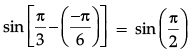= 1

Q.2: The value of k (k < 0) for which the function f defined as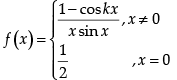is continuous at x = 0 is:
(a) ± 1
(b) –1
(c) ±1/2
(d) 1/2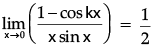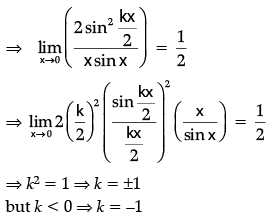Q.3: If A = [αij] is a square matrix of order 2 such that αij =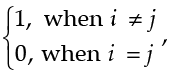then Ais:
(a)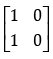(b)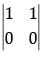(c)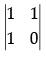(d)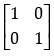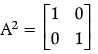Q.4: Value of k, for which A =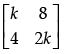is a singular matrix is:
(a) 4
(b) –4
(c) ±4
(d) 0

As A is singular matrix
⇒ |A| = 0

⇒ 2k2 – 32 = 0

⇒ k = ±4

Q.5: Find the intervals in which the function f given by f(x) = x2 – 4x + 6 is strictly increasing:
(a) (– ∞, 2) ∪ (2,
)
(b) (2,  ∞)
(c) (− ∞, 2)
(d) (– ∞, 2] ∪ (2,  ∞)

f(x) = x2 – 4x + 6
f'(x) = 2x – 4
Let f(x) = 0
⇒ x = 2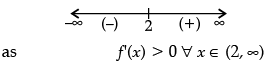⇒  f(x) is Strictly increasing in (2, ∞)

Q.6: Given that A is a square matrix of order 3 and | A | = - 4, then | adj A | is equal to:
(a) –4
(b) 4
(c) –16
(d) 16

as |adj A| = |A|n – 1,
where n is order of matrix A
= (–4)2 = 16

Q.7: A  relation R in set A = {1, 2, 3} is defined as R = {(1, 1), (1, 2), (2, 2), (3, 3)}. Which of the following ordered pair in R shall be removed to make it an equivalence relation in A
(a)
(1, 1)
(b)
(1, 2)
(c)
(2, 2)
(d)
(3, 3)

Q.8: If,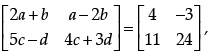then value of a + b – c + 2d is:

(a) 8

(b) 10

(c) 4

(d) –8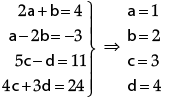∴ a + b – c + 2d = 8

Q.9: The point at which the normal to the curve y = x + 1/x, x > 0 x is perpendicular to the line 3x – 4y – 7 = 0 is:
(a) (2, 5/2)
(b) (±2, 5/2)
(c) (–1/2, 5/2)
(d) (1/2, 5/2)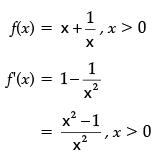As normal to f(x) is ⊥ to given line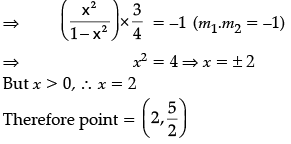Q.10: sin (tan–1x), where |x| < 1, is equal to:
(a)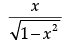(b)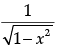(c)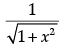(d)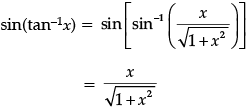Q.11: Let the relation R in the set A = {x ∈ Z : 0 ≤ x ≤ 12}, given by R = {(a, b) : |a – b| is a multiple of 4}. Then , the equivalence class containing 1, is:
(a)
{1, 5, 9}
(b)
{0, 1, 2, 5}

(c) ϕ

(d) A

Q.12: If ex + ey = ex+y, then dy/dx is:
(a) ey – x
(b) ex + y
(c) –ey – x
(d) 2e
x – y

ex + ey = ex + y
⇒ e–y + e–x = 1
Differentiating w.r.t. x: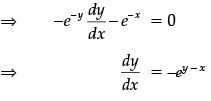Q.13: Given that matrices A and B are of order 3 × n and m × 5 respectively, then the order of matrix C = 5A + 3B is:
(a) 3 × 5 and m = n
(b) 3 × 5
(c) 3 × 3
(d) 5 × 5

Q.14: If y  = 5 cos x – 3 sin x, then d2y/dx2 is equal to:
(a) –y
(b) y
(c) 25y
(d) 9y

y = 5cos x – 3sin x
⇒ dy/dx = –5sin x – 3cos x

⇒ d2y/dx= –5cos x + 3sin x
= –y

Q.15: For matrix A =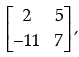(αdj A)' is equal to:
(a)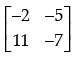(b)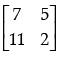(c)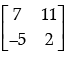(d)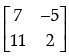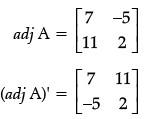Q.16: The points on the curve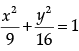at which the tangents are parallel to y-axis are:
(a) (0, ±4)
(b) (±4, 0)
(c) (±3, 0)
(d) (0, ±3)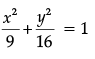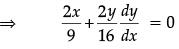⇒ Slope of normal =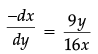As curve's tangent is parallel to y-axes

⇒ 9y/16x = 0
⇒ y = 0 and x = ±3
∴ points = (±3, 0)

Q.17: Given that A = [aij] is a square matrix of order 3 × 3 and |A| = −7, then the value of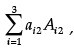where Aij denotes the cofactor of element αij is:
(a) 7

(b) –7

(c) 0

(d) 49

|A| = –7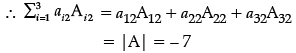Q.18: If y  = log(cos ex), then dy/dx is:
(a) cos ex−1
(b) e–x cos ex
(c) ex sin ex
(d) –ex tan e
x

y = log(cos ex)
Differentiating w.r.t. x: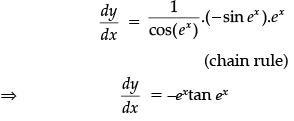Q.19: Based on the given shaded region as the feasible region in the graph, at which point(s) is the objective function Z = 3x + 9y maximum?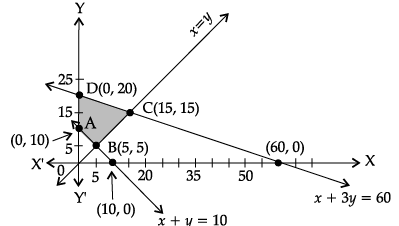(a) Point B
(b) Point C
(c) Point D
(d) every point on the line segment CD

Z is maximum 180 at points C (15, 15) and D(0, 20).
⇒ Z is maximum at every point on the line segment CD.

Q.20: The least value of the function f(x) = 2cos x + x in the closed interval [0, π/2] is:
(a) 2
(b) π/6 + √3
(c) π/2
(d) The least value does not exist

f(x) = 2cos x + x,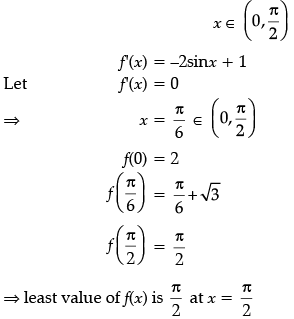Section - B

Q.21: Which of the following functions from Z into Z are bijections?
(a) f(x) = x3
(b) f(x) = x + 8
(c) f(x) = 4x + 1
(d) f(x) = x2 + 7

For bijection on Z, f(x) must be one-one and onto. Function f(x) = x2 + 7 is many-one as f(1) = f(–1) Range of f(x) = x3 is not Z for x ∈ Z. Also f(x) = 4x + 1 takes only values of type = 4k + 1 for x ∈ k ∈ Z But f(x) = x + 8 takes all integral values for x ∈ Z. Hence f(x) = x + 8 is a bijection of Z.

Q.22: If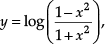then dy/dx is equal to
(a)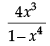(b)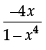(c)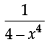(d)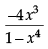Given that,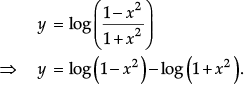Differentiate with respect to x, we have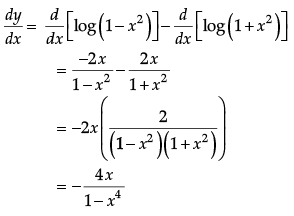Q.23: The feasible region for an LPP is always a _______ polygon.
(a) Concave
(b) Convex
(c) Both A and B
(d) None

The feasible region for an LPP is always a convex polygon.

Q.24: Local maximum of the function 3x 4 −20x3+36x 2 + 322 in (−10, 10) ?
(a) 2

(b) 3

(c) 0

(d) 5
Let f(x)
= 3x4 – 20x3 +36 x2 + 322
f '(x) = 12x3 – 60x2 + 72x
Put f '(x) = 0
12x3 – 60x2 + 72x = 0
12x (x2 – 5x + 6) = 0
12x (x – 2) (x – 3) = 0
x = 0, 2, 3
f ''(x) = 36x2 – 120x + 72
f ''(0) = 72 > 0
f ''(2) = 36 × 22 – 120 × 2 + 72 = –24 < 0
f ''(3) = 36 × 32 – 120 × 3 + 72 = 36 > 0
∴ We get local maxima at x = 2.

Q.25: If the matrix A is both symmetric and skew symmetric, then
(a) A is a diagonal matrix
(b) A is a zero matrix
(c) A is a square matrix
(d) None of these

If A is both symmetric and skew-symmetric then we have, A' = A and A' = –A
⇒ A = –A
⇒  A + A = 0
⇒ 2A = 0
⇒ A = 0
Therefore, A is a zero matrix.

Q.26: f(x) = [x] The function is:
(a) Not Continuous
(b) continuous
(c) Differentiable
(d) None of these

L.H.L and R.H.L. are not equal at integer points.

Q.27: What is the domain of the sec–1 x ?
(a) [−∞, ∞]
(b) (−∞, ∞)
(c) (–1, 1)
(d) (−∞, −1] ∪ [1,∞)

The sec function is periodic so to calculate its inverse function we need to make the function bijective. For that we have to consider an interval in which all values of the function exist and do not repeat. Now for the inverse of a function the domain becomes range and the range becomes domain. Thus the range of secant function, that is, (−∞,−1]∪[1,∞) becomes the domain of inverse function.

Q.28: If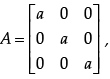then det (adj A) equals

(a) a27

(b) a9

(c) a6

(d) a2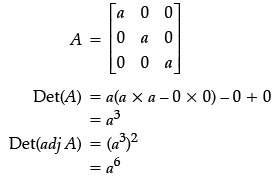Q.29: The tangent to the curve y = e2x at the point (0, 1) meets x-axis at :
(a) (0, 1)
(b) (-1/2, 0)
(c) (2, 0)
(d) (0, 2)

The equation of the curve is given by  y = e2x Since, it passes through the point (0, 1).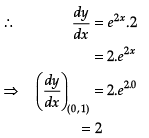= Slope of tangent to the curve.
∴ Equation of tangent is
y - 1 = 2(x -0)
⇒ y = 2x + 1
Since, tangent to the curve y = e2x at the point (0, 1) meets x-axis, i.e., y = 0.
∴ 0 = 2x + 1
x = -1/2
So, the required point is (-1/2, 0).

Q.30: Consider the set A = {1, 3, 5, 7}. The number of reflexive relations on set A is :
(a) 212
(b) 122
(c) 24
(d) 4
2

By definition, a relation in A is said to be reflexive if xRx, ∀x ∈ A.
So R is true.
The number of reflexive relations on a set containing n elements is 2 n2 -n
Here n = 4.
The number of reflexive relations on a set A = 212

Q.31: The interval on which the function f (x) = 2x+ 9x2 + 12x- 1 is decreasing is:
(a) [–1, ∞)
(b) (–2, –1)
(c) (–∞, –2]
(d) [–1, 1]

Given that,
f(x) = 2x3 + 9x+ 12x - 1
f'(x) = 6x2 + 18x + 12
= 6(x2 + 3x + 2)
6(x + 2)(x + 1)

So, f'(x) ≤ 0 for decreasing.
On drawing number lines as below: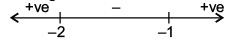We see that f '(x) is decreasing in (−2, −1).

Q.32: If A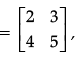Find A2

(a)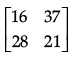(b)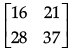(c)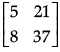(d) None of these

We need to find A2 and we know that A2 = A.A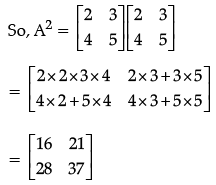Q.33: A corner point of a feasible region is a point in the region which is the __________ of two boundary lines.
(a) Intersection
(b) Disjoint
(c) Middle point
(d) None

A corner point of a feasible region is a point in the region which is the intersection of two boundary lines.

Q.34: The function f(x) = 2x3 – 3x2 – 12x + 4, has
(a) two points of local maximum
(b) two points of local minimum
(c) one maxima and one minima
(d) no maxima or minima

We have,
f(x) = 2x3 + 3x2 + 12x + 4
f'(x) = 6 x2 + 6x - 12
Now, f'(x) = 0
⇒ 6(x2 - x - 2) = 0
⇒ 6 (x + 1)(x - 2) = 0
⇒ x = -1 and x = +2
On number line for f’(x), we get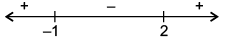Hence, x = −1 is point of local maxima and x = 2 is point of local minima.
So, f(x) has one maxima and one minima.

Q.35: If A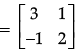then A2 – 5A + 7I2 is equal to
(a) 0
(b) 1
(c) –1
(d) 2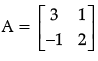Value of A2 – 5A + 7I2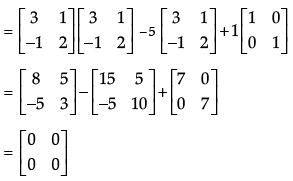Since, all the elements of matrix are zero. So, given matrix is null/zero matrix.

Q.36: The principal value of tan−1 (−√3) is:
(a) π/3
(b) - π/3
(c) 2π/3
(d) π/6

The principal value of tan−1 (−√3) means that we need to find an angle in the principal branch of the function where the tangent function is equal to -√3 . Hence the required value is - π/3.

Q.37: The principal value of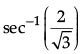is:
(a) π/3
(b) π/2
(c) π/6
(d) π/4

The principal value of means that we need to find an angle in the principal branch of the function where the secant function is equal to 2/√3. Hence the required value is π/6.

Q.38. Evaluate the determinant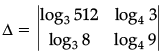(a) –17
(b) log3 18
(c) log28
(d) 15/2

For simplification usage of the properties of logarithm are required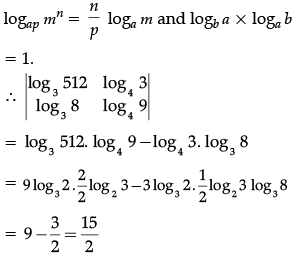Q.39: The points at which the tangents to the curve y = x3 – 12x + 18  are parallel to x-axis are:
(a) (2, 2), (–2, –34)
(b) (2, 34), (–2, 0)
(c) (0, 34), (–2, 0)
(d) (2, 2), (–2, 34)

The equation of the curve is given by
y = x3 – 12x + 18
On differentiating with respect to x, we get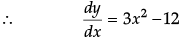So, the slope of line parallel to the x-axis,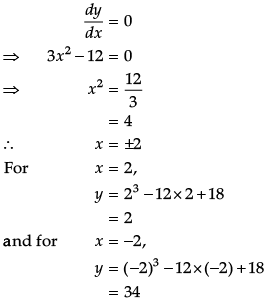So, the points are (2, 2) and (−2, 34).

Q.40: If matrix A = [1   2   3]. Write A.A' where A' is the transpose of matrix A.
(a) 4
(b) 21
(c) 14
(d) 28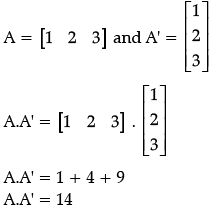Section - C

Q.41: The optimal value of the objective function is attained at the points.
(a) X-axis only
(b) Y-axis only
(c) Corner points of feasible region
(d) Origin only

The optimal value of the objective function is attained at the corner points of feasible region.

Q.42: For the curve y = 5x – 2x3, if x increases at the rate of 2 units/sec, then at x = 3 the slope of curve is changing at ________ units/sec.
(a) –72
(b) –36
(c) 24
(d) 48

Given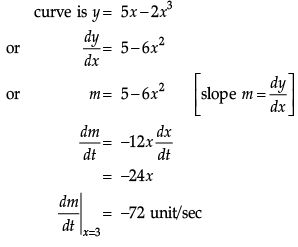Q.43: y = x(x – 3)2 decreases for the values of x given by:
(a) 1 < x < 3
(b) x < 0
(c) x > 0
(d) 0 < x < 3/2

Given that, y = x(x – 3)2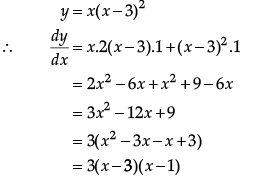So, y = x(x – 3)2 decreases for (1, 3).
[Since, y' < 0  for all x ∈ (1, 3), hence y is decreasing on (1, 3)].

Q.44: For the constraints of linear optimizing function Z = x1 + x2 given by x1 + x2 ≤ 1, 3x1 + x2 ≥1, there is feasible region
(a) Yes
(b) No
(c) Can't be determined
(d) None of these

Clearly from the graph below that there is no feasible region.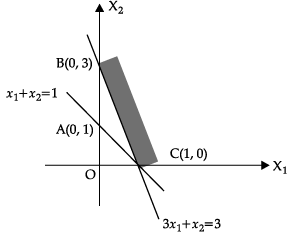Q.45: Let A =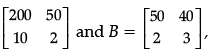then |AB| is equal to
(a) 460
(b) 2000
(c) 3000
(d) – 7000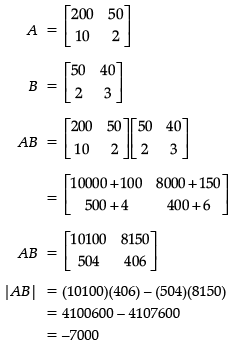CASE-STUDY

A manufacture produces three stationery products Pencil, Eraser and Sharpener which he sells in two markets. Annual sales are indicated below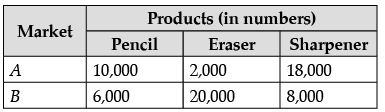If the unit Sale price of Pencil, Eraser and Sharpener are Rs 2.50, Rs 1.50 and Rs 1.00 respectively, and unit cost of the above three commodities are Rs 2.00, Rs 1.00 and Rs 0.50 respectively, then,
Based on the given information, answer the following questions.

Q.46: Total revenue of market A
(a) Rs 64,000
(b) Rs 60,400
(c) Rs 46,000
(d) Rs 40600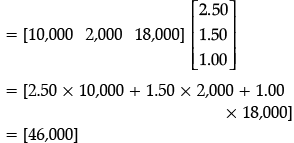Q.47: Total revenue of market B
(a) Rs 35,000
(b) Rs 53,000
(c) Rs 50,300
(d) Rs 30,500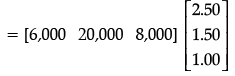= [2.50 × 6,000 + 1.50 × 20,000 + 1.00 × 8,000]
= [53,000]

Q.48: Cost incurred in market A
(a) Rs 13,000
(b) Rs 30,100
(c) Rs 10,300
(d) Rs 31,000

Cost incurred in marker A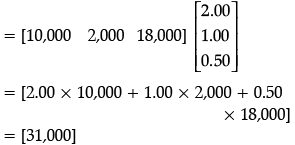Q.49: Profits in market A and B respectively are
(a) (Rs 15,000, Rs 17,000)
(b) (Rs  17,000, Rs 15,000)
(c) (Rs  51,000, Rs 71,000)
(d) (Rs 10,000, Rs 20,000)

Cost incurred in market B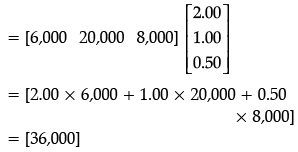Profit of market A & B = total revenue of A and B – Cost increased in market A and B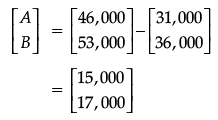Q.50: Gross profit in both markets
(a) Rs 23,000
(b) Rs 20,300
(c) Rs 32,000
(d) Rs 30,200

Gross profit in both markets = Profit in A + Profit in B
= 15,000 + 17,000
= Rs 32,000

The document Class 12 Mathematics: CBSE Sample Question Paper- Term I (2021-22)- 4 Notes | Study Mathematics (Maths) Class 12 - JEE is a part of the JEE Course Mathematics (Maths) Class 12.
All you need of JEE at this link: JEEUse Code STAYHOME200 and get INR 200 additional OFF

## Mathematics (Maths) Class 12

209 videos|209 docs|139 tests

Track your progress, build streaks, highlight & save important lessons and more!

,

,

,

,

,

,

,

,

,

,

,

,

,

,

,

,

,

,

,

,

,

;